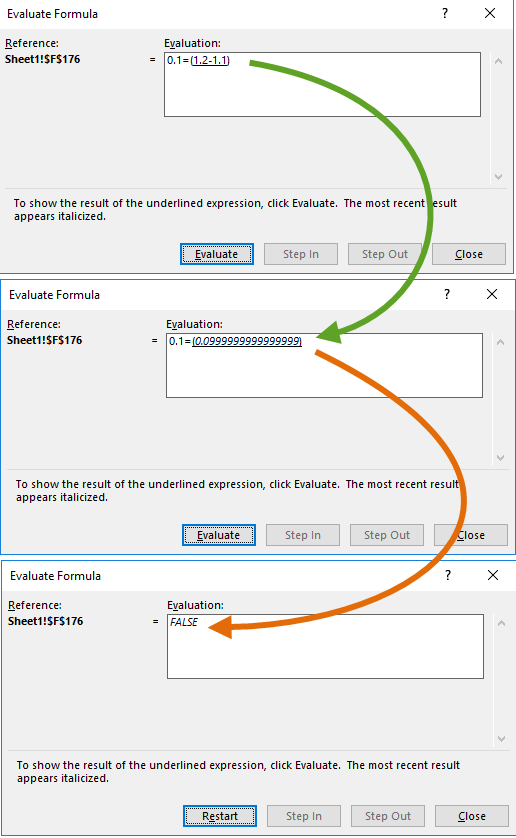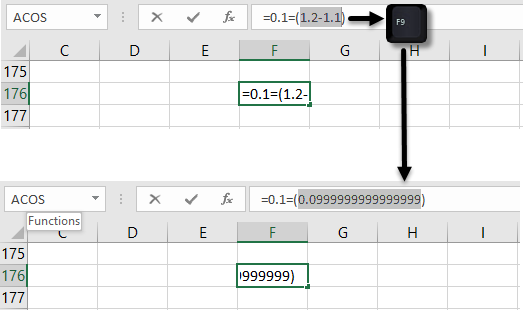# xlf QandA series

## Floating point precision, and cell formats

### QUESTION

WHY DOES THE LOGICAL EXPRESSION FORMULA =0.1=(1.2-1.1) RETURN FALSE?

### 1. Excel numbers and cell formats

• Excel numbers are stored and processed as Double Precision floating point numbers (64 bit)
• The numbers displayed in the cell have a number format. "General"" by default, except for Dates, Times, and Percentage
• The topic of Floating Point numbers is outside the scope of 90045

The short answer to the question is: because of the way floating point numbers are processed by a computer, an expression like 1.2-1.1 actually returns 0.0999999999999999 - 15 digits (ignoring the leading zeros and decimal indicator), rather than the expected value of 0.1. This does not happen in every instance, as illustrated by the examples in figure 4.

Several methods are displayed to illustrate this feature of Double Precision floating point numbers.

### 2. Using Evaluate Formula to display the floating point precision valueFig 2: Formula Evaluate dialog box[top panel] - the evaluate expression (the arithmetic expression in the brackets "()"), is shown by the underline. Press Evaluate to show the result of 1.2-1.1 [centre panel] - 1.2-1.1 returns 0.0999999999999999 - 15 digits (ignoring the leading zeros and decimal indicator). Press Evaluate to show the result of 0.1=0.0999999999999999 [bottom panel] - 0.1=0.0999999999999999 returns FALSE (Boolean)

### 3. Using F2 and F9 to display the floating point precision valueFig 3: Formula Edit, and F9 calculate[top panel] - with the formula cell as the active cell, press F2 to enter Edit mode (shown), then select the arithmetic expression in the brackets () [bottom panel] - press F9 to display the result (return value) of the expression. Press ESC to discard the return expression in the formula, and show the return value FALSE